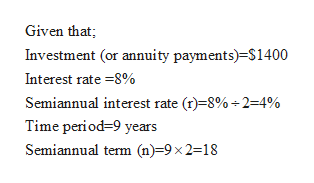# At the beginning of each year, Jerome invests \$1,400 semiannually at 8% for nine years. Using the tables found in the textbook, determine the cash value of the annuity due at the end of the ninth year.    A. \$37,939.86B. \$37,339.68C. \$37,399.68D. \$38,739.68

Question
33 views
At the beginning of each year, Jerome invests \$1,400 semiannually at 8% for nine years. Using the tables found in the textbook, determine the cash value of the annuity due at the end of the ninth year.

 A. \$37,939.86 B. \$37,339.68 C. \$37,399.68 D. \$38,739.68
check_circle

Step 1

Annuity due refers to repeating payments that are made at the beginning of each period. We can determine the cash value of the annuity due at the end of the ninth year using the steps below:

Step 2help_outlineImage TranscriptioncloseGiven that; Investment (or annuity payments)=\$1400 Interest rate =8% Semiannual interest rate (r)=8% -2=4% Time period=9 years Semiannual term (n)=9x 2=18 fullscreen
Step 3

Determination of cash value of annuity due us...

### Want to see the full answer?

See Solution

#### Want to see this answer and more?

Solutions are written by subject experts who are available 24/7. Questions are typically answered within 1 hour.*

See Solution
*Response times may vary by subject and question.
Tagged in